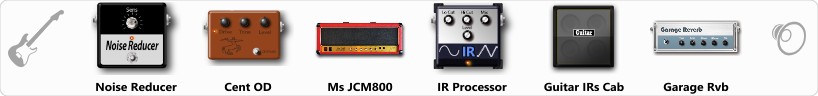# Shatarov Igor

Discussion in 'ToneLib-GFX presets' started by Shatarov Igor, Jan 30, 2020.

1. Metal Rhythm
Preset name: Metal Rhythm

Effects chain:Effect: "Noise Reducer" (Dynamics / Filter), active - "yes"
"Sens" = 81
"Mode" = Soft

Effect: "Cent OD" (Overdrive / Distortion), active - "yes"
"Drive" = 52
"Tone" = 70
"Level" = 74

Effect: "Ms JCM800" (Amp simulators), active - "yes"
"Gain" = 100
"Bass" = 82
"Middle" = 23
"Treble" = 39
"Presence" = 65
"Master" = 78
"Output" = 45
"Level (dB)" = 0

Effect: "IR Processor" (Dynamics / Filter), active - "yes"
"IR" = BBIRD 9100A 1960A P3_dc
"Low Cut (Hz)" = 111
"Hi Cut (kHz)" = 20.0
"Mix" = 100
"Level (dB)" = 0

Effect: "Guitar IRs Cab" (Cabinets), active - "yes"
"Model" = Peavey 5150 (4x12")
"Mic Position" = Center
"Mic Distance" = Near
"Low Cut (Hz)" = 60
"Hi Cut (kHz)" = 20.0
"Mix" = 41
"Level (dB)" = 0

Effect: "Garage Rvb" (Reverb), active - "yes"
"Time" = 5.2
"PreDelay" = 46
"LoDamp" = 0
"HiDamp" = 30
"Mix" = 10

Note: You will need to download and install the ToneLib-GFX software to use the preset.

File size:
54.6 KB
Views:
3,054